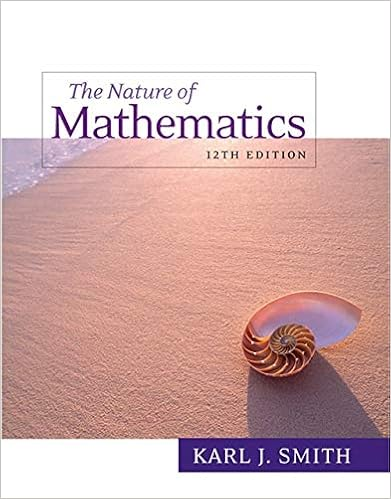# A horticulturist is investigating the phosphorous

• 56
• 50% (2) 1 out of 2 people found this document helpful

This preview shows page 25 - 28 out of 56 pages.

##### We have textbook solutions for you!
The document you are viewing contains questions related to this textbook.The document you are viewing contains questions related to this textbook.
Chapter 13 / Exercise 35
Nature of Mathematics
SmithExpert Verified
24. A horticulturist is investigating the phosphorous content of tree leaves from three differ-ent varieties of apple trees. Phosphorous content is known to follow a normal distributionfor each of the three varieties. Random samples of four leaves from each of the threevarieties are analyzed for phosphorous content. Population variances are known to beequal for all three varieties. We would like to test the hypothesis of equal populationmeans for the three varieties at the 10% level of significance. The critical value for theappropriate hypothesis test is:(A) 1.36(B) 2.81(C) 3.01(D) 5.22(E) 9.38
25. We take simple random samples from two normally distributed populations and measurethe value of some variableX.We conduct a pooled two-samplettest to determinewhether there is evidence that the population means differ. We calculate a test statisticoft= 2.47 and a P-value of 0.04. Suppose we had instead conducted the test using ananalysis of variance. What would be the value of the test statistic and the P-value?
26. We are conducting an analysis of variance to compare the means of four populations. Inconducting this test, we will commit a Type I error if we:
##### We have textbook solutions for you!
The document you are viewing contains questions related to this textbook.The document you are viewing contains questions related to this textbook.
Chapter 13 / Exercise 35
Nature of Mathematics
SmithExpert Verified
27. We would like to compare the means of three normally distributed populations withcommon variance. We conduct three separate pooled two-samplettests and we obtainthe P-values shown below:Test 1Test 2Test 3H0:μ1=μ2H0:μ1=μ3H0:μ2=μ3Ha:μ16=μ2Ha:μ16=μ3Ha:μ26=μ3P-value = 0.02P-value = 0.23P-value = 0.11Suppose we were to conduct an analysis of varianceFtest at the 5% level of significanceto compare the three means. Which of the following statements is true?
The nextthreequestions (28to30) refer to the following:A medical researcher wants to compare three different techniques designed to lower bloodpressure in patients with hypertension. Five subjects are assigned to each of the threegroups. The first group exercises, the second group meditates and the thrid group takes
•••